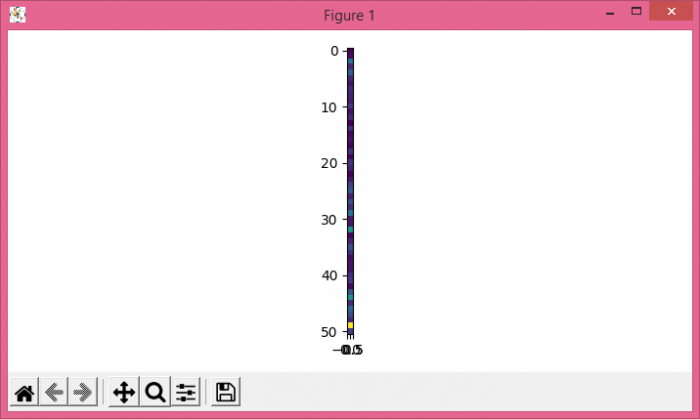# How to convert a .wav file to a spectrogram in Python3?

To convert a .wav file to a spectrogram in python3, we can take the following steps −

• Load a .wav file from local machine.

• Compute a spectrogram with consecutive Fourier transforms using spectrogram() method.

• Create a pseudocolor plot with a non-regular rectangular grid using pcolormesh() method.

• Use imshow() method with spectrogram.

• To display the figure, use show() method.

## Example

import matplotlib.pyplot as plt
from scipy import signal
from scipy.io import wavfile
plt.rcParams["figure.figsize"] = [7.00, 3.50]
plt.rcParams["figure.autolayout"] = True
plt.show()